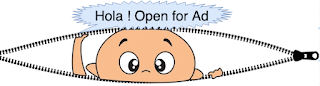## Sum of nodes at each level of binary tree in Java

Topics covered 1. Sum of nodes at each level of binary tree 2. Complete sample program Problem Statement :- Find sum of nodes at eac...Read More

## Zigzag level order traversal of binary tree in Java

Problem statement:- Traverse given binary tree alternatively left to right and right to left.In following diagram, LtoR represents : visit n...Read More

## Find sum of all elements of binary tree in Java - Iterative and recursive

Topics covered 1. Sum of nodes of binary tree -Iterative 2. Sum of nodes of binary tree -Recursive 3. Complete sample program Problem...Read More

## Find level with maximum sum in binary tree - display nodes and sum both

Problem statement :- For a given binary tree, find level with maximum sum and display all nodes at level level. For above binary tree: m...Read More